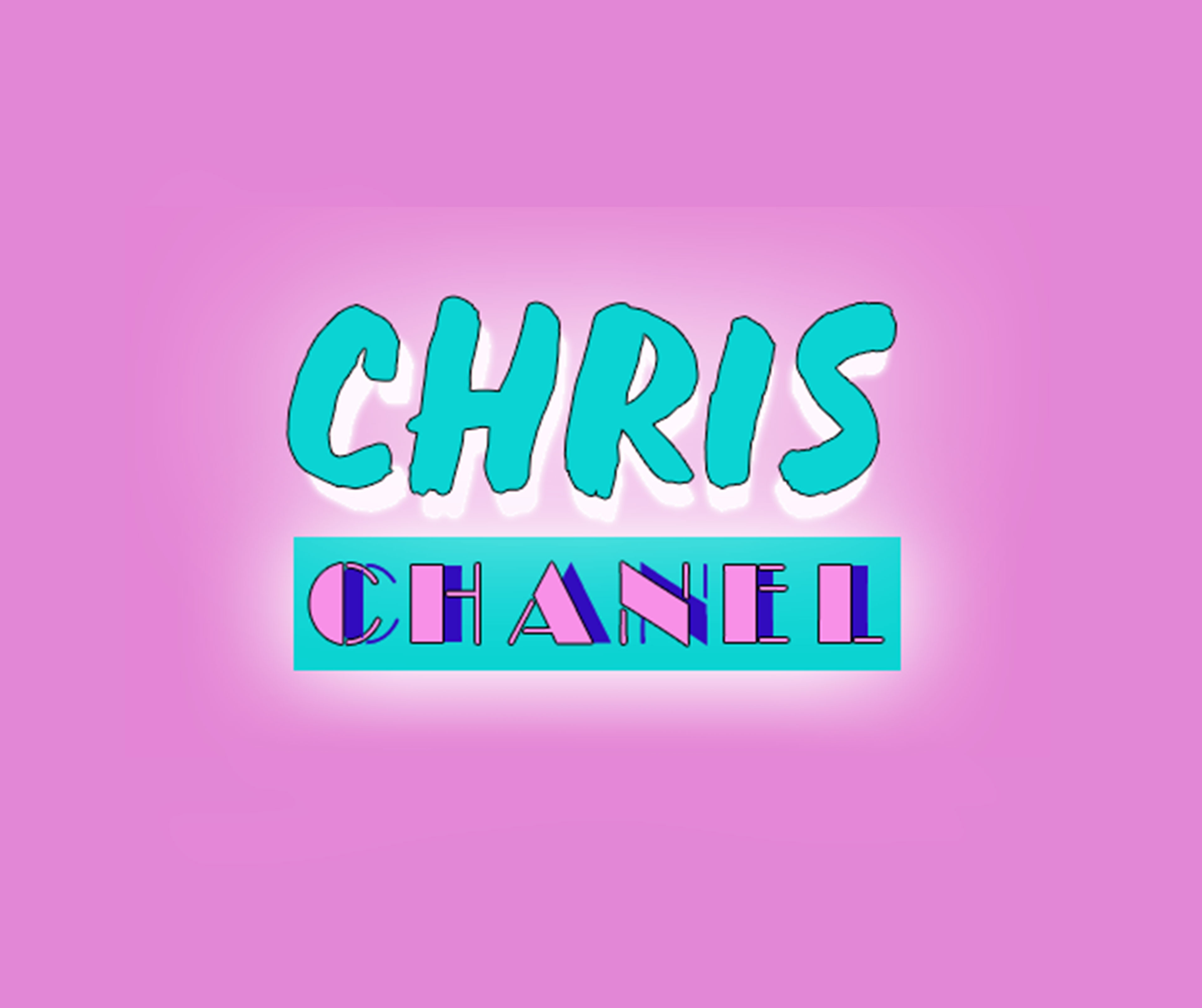# 2 Finger Ring

Regular price \$0.00 \$130.00 Sale

• ONLY ONE LETTER WILL BE CAPITALIZED
• UP TO NINE LETTERS
• 3-4 WEEKS FOR THE TURNAROUND TIME
• NO REFUNDS OR EXCHANGES
• Jewelry is gold plated and not real gold

 Find a piece of string or strip of paper no wider than 3/4". 2 Wrap it around the base of the appropriate finger. 3 Use a pen to mark the point on the string/paper where it overlaps, forming a complete circle. 4 With a ruler, measure the length from the starting end of the string/paper to the pen mark. 5 Use this measurement and the chart to the right to determine

• Ring Size

4 = 1 13/16

4.5 = 1 7/8

5 = 1 15/16

5.5 = 2

6  = 2 1/16

6.5 = 2 1/8

7= 2 3/16

7.5 = 2 1/4

8 = 2 5/16

8.5 = 2 3/8

9 = 2 7/16

9.5 = 2 1/2

10 = 2 9/16

10.5 = 2 5/8

11 = 2 11/16

11.5 = 2 3/4

12 =  2 13/16

12.5 = 2 7/8

13=   2 15/16

Spin to winx